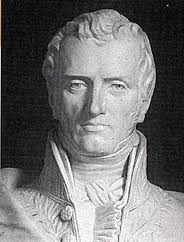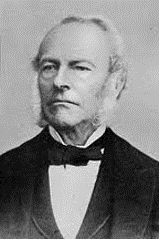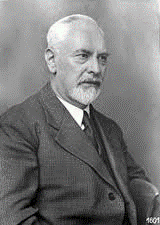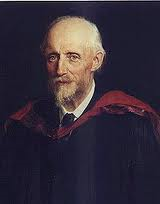# Mathematical modeling and numerical analysis of slow viscous currents in unbounded areas

### Содержание работы

Project Statement

Mathematical modeling and numerical analysis of slow viscous currents in unbounded areas

Lamtyugova Svetlana

Subject of the thesis

The subject of the thesis is mathematical models of slow viscous flows in unlimited domains and methods of their numerical analysis.

The subject of the thesis is an integral part of project entitled “Flow of superficial and underwater objects, ways of controlling the current past a body” pursued by the Hydromechanics Institute of Ukraine NAS.

The Background of the Problem

The concept fluid motion originated in ancient times, in ancient Greece.The first endeavour of defining the notion in question traces back to Aristotle who was the first to consider the water as a continuous environment.Archimedes formulated the laws of buoyancy and applied them to floating bodies and submerged ones.Leonardo da Vinci (1452-1519) derived the equation of conservation of mass in one-dimensional steady flow.Isaac Newton (1642-1727) formulated the laws of motion and the law of viscosity of the linear fluids.Daniel Bernoulli * introduced the concept hydromechanics ** established the dependence between the specific energies of the fluid motion *** investigated into the problem concerning the pressure of the liquid jet on a plate.

Leonhard Euler * derived the equations of liquids and gases equilibrium and motion ** formulated the law of liquid mass conservation. *** treated some motion issues in relation to the empirical problems of shipbuilding and the construction of hydraulic machines.Henri Navier (1785 - 1836) obtained the equations of motion of viscous liquid.Immense contribution to the elucidation of these equations has been made by Stokes.

The Navier–Stokes equations model the flow of a fluid with positive viscosity.

Ludwig Prandtl (1875 - 1953) the conception of an asymptotic boundary layer: “with low-viscosity region of fluid flow can be divided into two parts: the inner area in which the viscosity can be neglected, and a thin boundary region (Boundary layer), in which the fluid flows parallel to the boundaries and the fluid velocity drops to zero as it approaches the edge of the area”O. Reynolds established general criterion of turbulence phenomenon emerging.

The approximate methods for solving the Navier-Stokes equations can be divided into two classes: the finite-difference methods (net method) and the approximate analytical methods (the Galerkin methods, the least squares method, etc.).

The various relaxation procedures are used.

The fictitious domain method in conjunction with a process of successive approximations is applied.

One of the most widely used and efficient numerical methods in fluid mechanics is the finite element method.

The disadvantage of these methods is the substitution of the complicate field on simple approximate area.

Free from this disadvantage is the method of R-functions developed by the school of V. L. Rvachev, Ukraine NAS academic. It takes into account the geometry of the area and boundary conditions of the problem.

The aim of the thesis

is to simulate the mathematical modeling methods of slow viscous currents in unbounded areas.

* To develop and substantiate the method of solving the problem of estimating the slow flow of viscous fluid (the Stokes approximation) in a simply and multiply connected areas.

** To develop a method for solving the biharmonic equation for the stream function taking into consideration the necessity to pursue the Stokes task.

*** To investigate the convergence of the methods to be obtained.

**** To pursue numerical simulations and perform software implementation of the methods and algorithms to be developed.

***** To apply the numerical methods to be developed for solving model problems via calculating the motion of a viscous incompressible fluid flow past bodies in the presence of an external magnetic field.

Methods

(i) the methods of functional analysis and mathematical physics to prove the convergence;

(ii) the Galerkin-Petrov projection method and the method of successive approximations for the solution of nonlinear equations;

(iii) the Gauss quadrature formulas for numerical integration;

(iv) linear algebra methods for solving systems of linear algebraic equations;

(v) mathematical instrument of the theory of R-functions for the construction of normalized equations of the areas boundaries;

(vi) the method of R-functions for the construction of complete structures of boundary value problems;

(vii) the methods of spline approximations.

Assumption

The methods to be developed are assumed to describe the flows in question with minimal deviation.

Theoretical value of the problem

The methods to be developed in the thesis are certain to be of concern to a wide range of scholars having been involved in treating the issues in question. Moreover, they can also serve as the basis for the special courses in the universities.

The empirical value of the problem

The methods of plane viscous fluid calculation in simply and multiply connected areas, to be developed in the thesis, will enable making the effective numerical simulation of viscous incompressible fluid flows in the

areas of complex geometry. The results to be obtained are believed to enhance implementing computational experiments in mathematical modeling of various physical, mechanical, biological flows.

Тип:
Доклады
Размер файла:
320 Kb
Скачали:
0